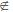×#### Thank you for registering.

One of our academic counsellors will contact you within 1 working day.

Click to Chat

1800-1023-196

+91-120-4616500

CART 0

• 0

MY CART (5)

Use Coupon: CART20 and get 20% off on all online Study Material

ITEM
DETAILS
MRP
DISCOUNT
FINAL PRICE
Total Price: Rs.

There are no items in this cart.
Continue Shopping```Coefficient of A Particular TermIn the expression of (a + x)n, the coefficient of second term is nC1, of the third term is nC2, of he fourth term is nC3 and so on. The suffix in each term being one less than the number of the term to which it applies; hence nCr is the coefficient of the (r+1)th term. This is called the General Term, because by giving different numerical values to r any of the coefficients may be found. Also, the indices of a and x in the (r + 1)th term are expressable in terms of r.

Thus, General term of the expansion (a + x)n is dented as

Tr+1 = nCr an-r xr = ((n(n-1)(n-2)....(n-r+1))/r!)  an-r xr.

Note: General term is useful in finding the following in Binomial expression.
(a) Particular term.
(b) Middle term
(c) Term independent of x.
(d) Term containing the greatest coefficient of x.

Illustration:

Find the fifth term in the expansion of (a + 2x3)17.

Solution:

General term of the expansion (a + x)n is

Tr+1 = nCr an-r xr.

Now, for the given expression, required term is

T5 = 17C4 a13 (2x3)4.

= 38080 a13 x12.

Illustration:

Find the term independent of x in (1 + x + 2x3)  ((3/2)x2 -  (1/3x))9.

Solution:

We want to find the term independent of x in product of (1 + x + 2x3) and  ((3/2)x2 -  (1/3x))9 .  So, we should find the terms containing x0, x-1 and x-3 in the expansion  ((3/2)x2 -  (1/3x))9  and multiply them respectively with the appropriate term from (1 + x + 2x3) i.e. constant term i.e. 1, term containing x i.e. 1 and the term containing x3 i.e. 2.

Now, Tr+1 = nCr an-r xr [this is general term in (a+x)n]

= 9Cr ((3/2)x2)9-r (-(1/3x))r

This is general term in [((3/2)x2 - (1/3x))9]

(i) To find the term containing x0 we use 18 - 3r = 0, i.e. r = 6

so coefficient of x0 we use (-1)6 9C6 3-3 2-3 = 9C6  (1/22.33).

(ii) To find the term containing x-1 we use 18-3r = -1 i.e. r = 19/3 (I) which is impossible.

(iii) To find the term containing x-3 we put 18-3r = -3 i.e. r = 7. So, coefficient of x-3 in ((3/2)x2 - (1/3x))9 = (-1)7 9C7 3-5 2-2 = -9C7  (1/23.35) .

Therefore, required coefficient = 9C6 (1/23.33)× 1-2 × 9C7 (1/23.35) = 17/54.

Illustration:

Find the coefficient of x24 in  (x2 +3a/x)15.

Solution:

The general term ((r + 1)th term) is

(x2 +3a/x)15 = 15Cr(x2)15-r (3a/x)r = 15Cr x30-2r  (3rar/xr) = 15Cr 3r ar x30-3r

If this term contains x24, then 30-3r = 24

=> 3r = 6 => r = 2.

Therefore, the coefficient of x24 = 15C2 9a2.

Illustration:

If the binomial co-efficient of the (2r + 4)th term and the (r - 2)th term in the expansion of (1 + x)18 are equal, find the value r.

Solution:

The coefficient of (2r + 4)th term in (1 + x)18 = 18C2r+3 and the coefficient of (r-2)th term = 18Cr-3, so that 18C2r+3 = 18Cr-3.

Either 2r + 3 = r - 3, => r = -6,

Or 2r + 3 + r - 3 = 18 => 3r = 18 => r = 6.             (Since r ε N)

Illustration:

If the 4th term in the expansion (px + 1/x)n is independent of x, find the value of n. Also calculate p if the 4th term is 5/2.

Solution:

Here T4 = T3+1 = nC3(px)3 (1/x)n-3 = nC3 p3 x6-n.

T4 is independent of x => 6 - n = 0 or n = 6.

Now given T4 = 5/2 => 6C3. p3 = 5/2 => p3 = 5/2 .= 1/8 => p = 1/2.

Illustration:

If the expansion of (1 + x)43 the coefficient of (2r + 1)th term is equal to the  coefficient of (r + 2)th term find r.

Solution:

Given in the expansion of (1 + x)43 the coefficient of (2r + 1)th term = the coefficient of (r + 1)th term.

43C2r = 43Cr+1. Either 2r = r + 1 => r = 1,

or 2r + r + 1 = 43 => r = 14.

Hence r =1, 14

Illustration:

Find the coefficient of x3 in the expansion of (1 + x + 2x2) (2x2 - (1/3x))9.

Solution:

Tr+1 in  (2x2 - (1/3x))9 is 9Cr (2x2)9-r (-1/3x)r = 9Cr29-r (-1/3)r x18-3r

=> coefficient of x3 is 9C5.24 (-1/3)5 = -224/7.

IIT JEE and JEE Main /Advanced (known as AIEEE till 2012) study material is available online free of cost at askIITians.com. Study Physics, Chemistry and Mathematics at askIITians website and be a winner. We offer numerous live online classroom courses as well for live online IIT JEE preparation - you do not need to travel anywhere any longer - just sit at your home and study for IIT JEE live online with askIITians.com

To read more, Buy study materials of Binomial Theorem comprising study notes, revision notes, video lectures, previous year solved questions etc. Also browse for more study materials on Mathematics here.
```### Course Features

• 731 Video Lectures
• Revision Notes
• Previous Year Papers
• Mind Map
• Study Planner
• NCERT Solutions
• Discussion Forum
• Test paper with Video Solution